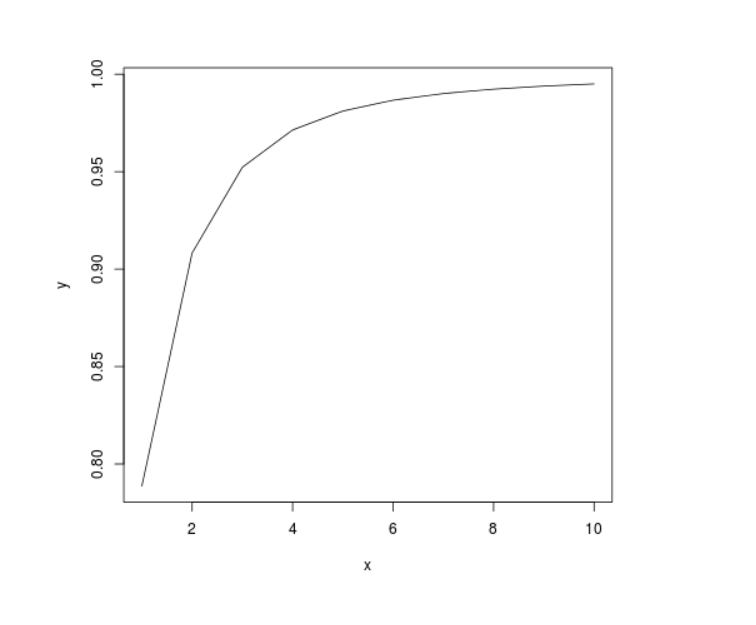GeeksforGeeks App
Open AppBrowser
Continue

# Perform the Probability Cumulative Density Analysis on t-Distribution in R Programming – pt() Function

`pt()` function in R Language is used to return the probability cumulative density of the Student t-distribution.

Syntax: pt(x, df)

Parameters:
x: Random variable
df: Degree of Freedom

Example 1:

 `# R Program to perform``# Cumulative Density Analysis`` ` `# Calling pt() Function``pt(``2``, ``10``)``pt(.``542``, ``15``)`

Output:

``` 0.963306
 0.7021105
```

Example 2:

 `# R Program to perform``# Cumulative Density Analysis`` ` `# Creating a vector``x <``-` `seq(``1``, ``10``, by ``=` `1``)`` ` `# Calling pt() Function``y <``-` `pt(x, ``2``)`` ` `# Plotting probability distribution graph``plot(x, y, ``type` `=``"l"``)`

Output:My Personal Notes arrow_drop_up